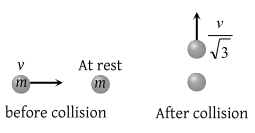A tennis ball is released from height h above ground level. If the ball makes inelastic collision with the ground, to what height will it rise after third collision

(1) he6

(2) e2h

(3) e3h

(4) None of these

Concept Questions :-

Linear momentum
High Yielding Test Series + Question Bank - NEET 2020

Difficulty Level:

A mass 'm' moves with a velocity 'v' and collides inelastically with another identical mass. After collision the 1st mass moves with velocity $\frac{v}{\sqrt{3}}$in a direction perpendicular to the initial direction of motion. Find the speed of the 2nd mass after collision(1) $\frac{2}{\sqrt{3}}v$

(2) $\frac{v}{\sqrt{3}}$

(3) v

(4) $\sqrt{3}\text{\hspace{0.17em}}v$

Concept Questions :-

Linear momentum
High Yielding Test Series + Question Bank - NEET 2020

Difficulty Level:

A sphere collides with another sphere of identical mass. After collision, the two spheres move. The collision is inelastic. Then the angle between the directions of the two spheres is

(1) 90°

(2) 0°

(3) 45°

(4) Different from 90°

Concept Questions :-

Linear momentum
High Yielding Test Series + Question Bank - NEET 2020

Difficulty Level:

A particle of mass m moving eastward with a speed v collides with another particle of the same mass moving northward with the same speed v. The two particles coalesce on collision. The new particle of mass 2m will move in the north-easterly direction with a velocity

(1) v/2

(2) 2v

(3) $v/\sqrt{2}$

(4) v

Concept Questions :-

Linear momentum
High Yielding Test Series + Question Bank - NEET 2020

Difficulty Level:

A bullet of mass a and velocity b is fired into a large block of mass c. The final velocity of the system is

(1) $\frac{c}{a+b}\cdot b$

(2) $\frac{a}{a+c}\cdot b$

(3) $\frac{a+b}{c}.a$

(4) $\frac{a+c}{a}\cdot b$

Concept Questions :-

Linear momentum
High Yielding Test Series + Question Bank - NEET 2020

Difficulty Level:

A bag (mass M) hangs by a long thread and a bullet (mass m) comes horizontally with velocity v and gets caught in the bag. Then for the combined (bag + bullet) system

(1) Momentum is $\frac{mvM}{M+m}$

(2) Kinetic energy is $\frac{m{v}^{2}}{2}$

(3) Momentum is $\frac{mv\left(M+m\right)}{M}$

(4) Kinetic energy is $\frac{{m}^{2}{v}^{2}}{2\left(M+m\right)}$

Concept Questions :-

Linear momentum
High Yielding Test Series + Question Bank - NEET 2020

Difficulty Level:

A particle of mass m moving with velocity v strikes a stationary particle of mass 2m and sticks to it. The speed of the system will be

(1) v/2

(2) 2v

(3) v/3

(4) 3v

Concept Questions :-

Linear momentum
High Yielding Test Series + Question Bank - NEET 2020

Difficulty Level:

A moving body of mass m and velocity 3 km/h collides with a rest body of mass 2m and sticks to it. Now the combined mass starts to move. What will be the combined velocity

(1) 3 km/h

(2) 2 km/h

(3) 1 km/h

(4) 4 km/h

Concept Questions :-

Linear momentum
High Yielding Test Series + Question Bank - NEET 2020

Difficulty Level:

If a skater of weight 3 kg has initial speed 32 m/s and second one of weight 4 kg has 5 m/s. After collision, they have speed (couple) 5 m/s. Then the loss in K.E. is

(1) 48 J

(2) 96 J

(3) Zero

(4) None of these

Concept Questions :-

Linear momentum
High Yielding Test Series + Question Bank - NEET 2020

Difficulty Level:

A metal ball of mass 2 kg moving with a velocity of 36 km/h has an head on collision with a stationary ball of mass 3 kg. If after the collision, the two balls move together, the loss in kinetic energy due to collision is

(1) 40 J

(2) 60 J

(3) 100 J

(4) 140 J

Concept Questions :-

Linear momentum